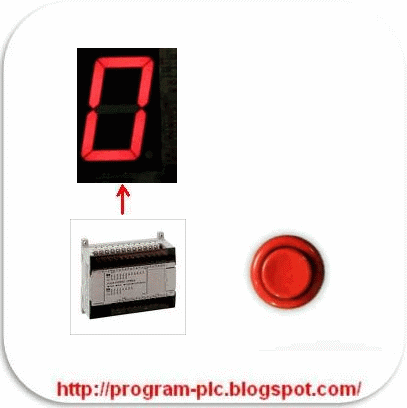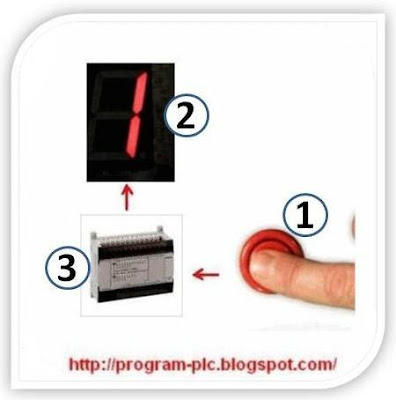## Display Seven Segment with PLC

Simulation Display Seven SegmentDetail Display Seven Segment with PLCInformation on Drawing Numbers for Display Seven Segment with PLC :
1. Push Button Switch ( without lock )
2. One Display Seven Segment
3. PLC or Programmable Logic Controller

PLC Wiring Diagram for 7 Segment :
1. common anode seven segment to plc
2. common cathode seven segment to plc

Number Of Inputs and Output PLC applied :
1. Number Of Inputs PLC is 1 Input :
--- 1 Unit Input for Push Button Switch ( without lock ).
--- Total Number Of Inputs PLC is Minimum 1 Input Unit.

2. Number Of Output PLC is 7 Output :
--- 7 Unit Output for Seven Segment.
--- Total Number Of Outputs PLC is Minimum 7 Output Unit.

Diagram 7 Segment and PLC OutputNumeric Seven Segment from 0 to 9Sequence PLC Programming for Display Seven Segment :

Numeric Display 0
0. If Data Memory = 0 Then Display Seven Segment = 0. PLC OUTPUT : OUT0=ON, OUT1=ON, OUT2=ON, OUT3=ON, OUT4=ON, OUT5=ON, OUT6=OFF
Numeric Display 1
1. If Push Button Switch = ON (Push-1) Then Data Memory = 1 And Display Seven Segment = 1.
PLC OUTPUT : OUT0=OFF, OUT1=ON, OUT2=ON, OUT3=OFF, OUT4=OFF, OUT5=OFF, OUT6=OFF

Numeric Display 2
2. If Push Button Switch = ON (Push-2) Then Data Memory = 2 And Display Seven Segment = 2.
PLC OUTPUT : OUT0=ON, OUT1=ON, OUT2=OFF, OUT3=ON, OUT4=ON, OUT5=OFF, OUT6=ON

Numeric Display 3
3. If Push Button Switch = ON (Push-3) Then Data Memory = 3 And Display Seven Segment = 3.
PLC OUTPUT : OUT0=ON, OUT1=ON, OUT2=ON, OUT3=ON, OUT4=OFF, OUT5=OFF, OUT6=ON

Numeric Display 4
4. If Push Button Switch = ON (Push-4) Then Data Memory = 4 And Display Seven Segment = 4.
PLC OUTPUT : OUT0=OFF, OUT1=ON, OUT2=ON, OUT3=OFF, OUT4=OFF, OUT5=ON, OUT6=ON

Numeric Display 5
5. If Push Button Switch = ON (Push-5) Then Data Memory = 5 And Display Seven Segment = 5.
PLC OUTPUT : OUT0=ON, OUT1=OFF, OUT2=ON, OUT3=ON, OUT4=OFF, OUT5=ON, OUT6=ON

Numeric Display 6
6. If Push Button Switch = ON (Push-6) Then Data Memory = 6 And Display Seven Segment = 6.
PLC OUTPUT : OUT0=ON, OUT1=OFF, OUT2=ON OUT3=ON, OUT4=ON, OUT5=ON, OUT6=ON

Numeric Display 7
7. If Push Button Switch = ON (Push-7) Then Data Memory = 7 And Display Seven Segment = 7.
PLC OUTPUT : OUT0=ON, OUT1=ON, OUT2=ON OUT3=OFF, OUT4=OFF, OUT5=OFF, OUT6=OFF

Numeric Display 8
8. If Push Button Switch = ON (Push-8) Then Data Memory = 8 And Display Seven Segment = 8.
PLC OUTPUT : OUT0=ON, OUT1=ON, OUT2=ON OUT3=ON, OUT4=ON, OUT5=ON, OUT6=ON

Numeric Display 9
9. If Push Button Switch = ON (Push-9) Then Data Memory = 9 And Display Seven Segment = 9.
PLC OUTPUT : OUT0=ON, OUT1=ON, OUT2=ON, OUT3=ON, OUT4=OFF, OUT5=ON, OUT6=ON

Numeric Display 0
0. If Push Button Switch = ON (Push-10) Then Data Memory = 0 And Display Seven Segment = 0. PLC OUTPUT : OUT0=ON, OUT1=ON, OUT2=ON, OUT3=ON, OUT4=ON, OUT5=ON, OUT6=OFF

Table Numeric Seven Segment versus PLC OutputPlease Click : Display Seven Segment

Can You make Program Ladder PLC ?

If Can't :

Seven Segment Display With PLC Omron

Seven Segment Display With PLC Mitsubishi

Seven Segment Display With PLC Keyence

Labels:

#### You may also like these ebook:

Get Free PLC eBook directly sent to your email,
and email subscription to program-plc.blogspot.com

We hate SPAM. Your information is never sold or shared with anyone.

Your Email Will Be 100% Secured !